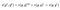# The BLS Signature Scheme — A short intro

Outline:

1. What is a Signature Scheme?
2. Some well-known signature schemes
3. Diffie-Hellman Problem (DHP)
4. Computational DHP vs Decisional DHP
5. Gap Group
6. BLS Signature Scheme

A digital signature scheme is essentially a mathematical setup to prove that a message has been authenticated by the sending party. The signature scheme ensures:

• Message is authenticated (by sender)
• Sender cannot deny sending the message (non-repudiation)
• Message is not tampered with (data integrity)

Diffie and Hellman conjectured the existence of a digital signature scheme in 1976 based on trapdoor one-way functions. (One-way functions have not been proven to exist to date.) Shortly after, the RSA encryption scheme was invented based upon the assumption that the factorization of semi-primes (N=p*q, where p, q are primes) is a difficult problem.

Soon after, many more encryption schemes were invented:

Some prominent encryption schemes in literature and the fundamental reasoning behind their formulation include:

• Boneh — Lynn — Shacham (BLS): Based on the existence of random oracles and the intractability of the computational Diffie-Hellman problem in a gap Diffie-Hellman group.
• ElGamal: Based on the hardness of the discrete logarithm problem.
• ECDSA: Based on the fact that given a point A on an elliptic curve and another point P = n*A, it is difficult to find n.
• Ring signature: Any non-empty subset of a group of n people can sign a message without the identity of any of the participants being leaked. It is a signature scheme built on other schemes.
• RSA: Factorization of numbers (semi-primes in particular) is hard.
• Schnorr Signature: Based on the hardness of the discrete logarithm problem.

With the broad understanding above, we now take a look at the Diffie-Hellman problem.

# Diffie-Hellman Problem (DHP)

Given a multiplicative group G and a generator g, the Diffie-Hellman Problem (DHP) asks whether it is easy to find g^xy given g, g^x, and g^y, where x, y are some randomly chosen integers. Solving the Diffie-Hellman problem (DHP) in many groups has been shown to be almost as hard as the discrete log problem (DLP).

# Computational DHP (CDHP) vs Decisional DHP (DDHP)

At this stage, it is important to make a distinction between the computational DHP and the decisional DHP. The computational DHP involves finding g^xy from g^x and g^y, as described before, whereas the decisional DHP (DDHP) involves distinguishing g^xy from a random group element given g, g^x, g^y. It is trivial to see that the computational DHP is always as difficult as the decisional DHP, but it is unclear to see if one is strictly tougher in general.

# Gap Group

A gap group is a group in which the computational DHP is difficult but the DDHP can be efficiently solved. One particular example is that of non-degenerate, efficiently computable, bilinear pairings. A pairing in cryptography is defined as follows:

We can see that the decisional DHP when a bilinear pairing is defined is easy because given g^x, g^y, g^z, where z = x*y, we can verify whether g^z is different from a random element in the group G as follows:DDHP is easy in a group over which a bilinear pairing is defined

However, the computational DHP is conjectured to be intractable in this instance.

# BLS Signature Scheme

Having given a primer to digital signature schemes and some basic background, the following are the components of the BLS signature scheme:

• Bilinear Pairing e( · , · )
• Elliptic Curve Group elements as signatures

Assumptions:

• Existence of random oracle
• Intractability of CDHP in a gap DH group

The scheme:

• Generate: Select a number x as your private key in the range [0, r-1]. The public key shared is g^x
• Given the private key x and some message m, the signature is given by (H(m))^x
• To verify that the signature is valid, we check that e((H(m))^x,g) = e(H(m), g^x), given g^x, g

Note that the above signature scheme works, because we have a bilinear pairing on a group and we cannot find the private key x given g^x and g.

In future posts, we will go deeper into the use of digital signature schemes in cryptocurrencies and blockchains.

PhD @ Courant Institute of Mathematical Sciences. Interested in Machine Learning, Cryptography and Blockchain.